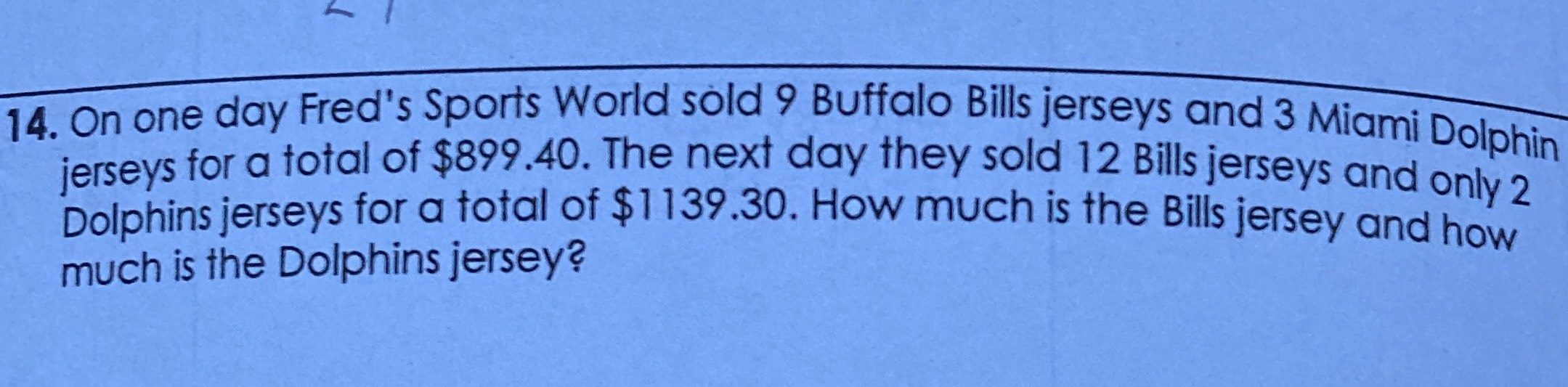### ¿Todavía tienes preguntas de matemáticas?

Pregunte a nuestros tutores expertos
Algebra
Pregunta14. On one day Fred's Sports World sold $$9$$ Buffalo Bills jerseys and $$3$$ Miami Dolphin jerseys for a total of $$\ 899.40$$ . The next day they sold $$12$$ Bills jerseys and only $$2$$ Dolphins jerseys for a total of $$\ 1139.30$$ . How much is the Bills jersey and how much is the Dolphins jersey?

$$b= 89.95\\m= 29.95$$